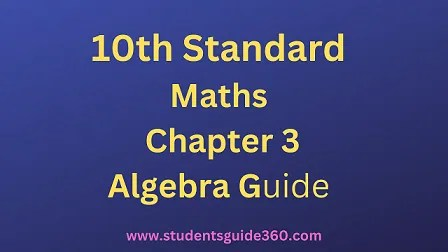# 10th Maths Chapter 3 Algebra Exercise 3.8 Guide

10th Standard Maths Chapter 3 Algebra Samacheer Kalvi Guide Exercise 3.8 Book Back Answers Solutions. TN 10th SSLC Samacheer Kalvi Guide. 10th All Subject Guide – Click Here. Class 1 to 12 All Subject Book Back Answers – Click Here## 10th Maths Chapter 3 Algebra Exercise 3.8 Guide

### 1. Find the square root of the following polynomials by division method

• (i) x4 – 12×3 + 42×2 – 36x + 9
• (ii) 37×2 – 28×3 + 4×4 + 42x + 9
• (iii) 16×4 + 8×2 + 1
• (iv) 121×4 – 198×3 – 183×2 + 216x + 144

Solution:
The long division method in finding the square root of a polynomial is useful when the degrees of a polynomial is higher.### 2. Find the square root of the expression x2y2−10xy+27−10yx+y2x2

Solution:### 2. Find the values of a and b if the following polynomials are perfect squares

• (i) 4×4 – 12×3 + 37×2 + bx + a
• (ii) ax4 + bx3 + 361ax2 + 220x + 100

Solution:
(i)Since it is a perfect square.
Remainder = 0
⇒ b + 42 = 0, a – 49 = 0
b = -42, a = 49

(ii) ax4 + bx3 + 361ax2 + 220x + 100Since remainder is 0
a = 144
b = 264

### 3. Find the values of m and n if the following expressions are perfect squares

(i) 1×4−6×3+13×2+mx+n
(ii) x4 – 8×3 + mx2 + nx + 16
Solution:
(i)(ii)Since the remainder is 0,
m = 24, n = -32